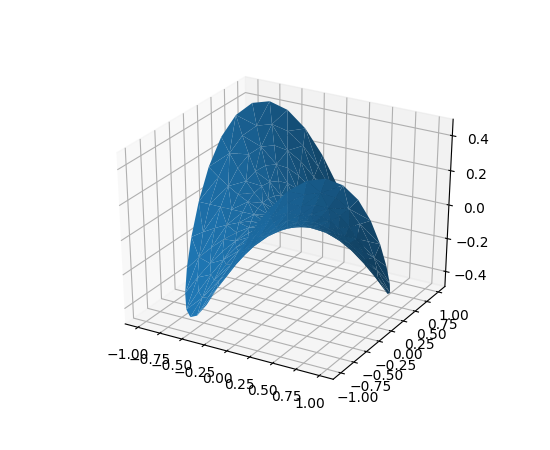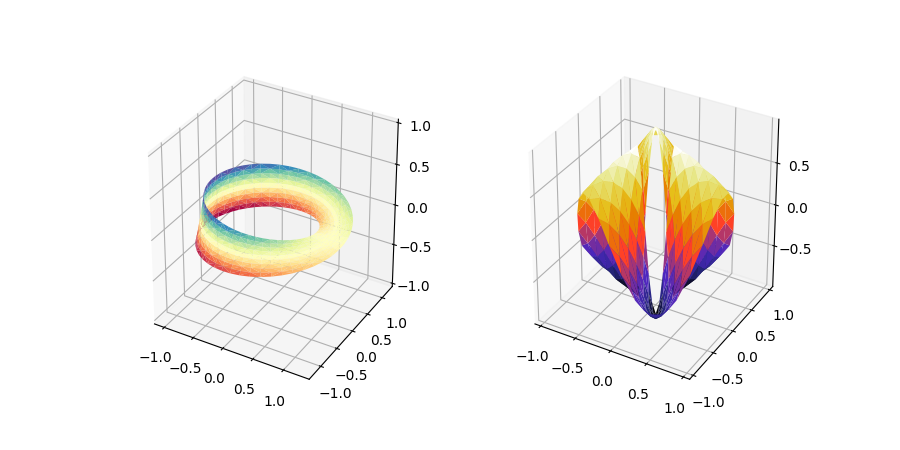# The mplot3d Toolkit¶

Generating 3D plots using the mplot3d toolkit.

## Getting started¶

An Axes3D object is created just like any other axes using the projection='3d' keyword. Create a new matplotlib.figure.Figure and add a new axes to it of type Axes3D:

import matplotlib.pyplot as plt
from mpl_toolkits.mplot3d import Axes3D
fig = plt.figure()


New in version 1.0.0: This approach is the preferred method of creating a 3D axes.

Note

Prior to version 1.0.0, the method of creating a 3D axes was different. For those using older versions of matplotlib, change ax = fig.add_subplot(111, projection='3d') to ax = Axes3D(fig).

### Line plots¶

Axes3D.plot(xs, ys, *args, zdir='z', **kwargs)[source]

Plot 2D or 3D data.

Argument Description
xs, ys x, y coordinates of vertices
zs z value(s), either one for all points or one for each point.
zdir Which direction to use as z ('x', 'y' or 'z') when plotting a 2D set.

Other arguments are passed on to plot()

### Scatter plots¶

Axes3D.scatter(xs, ys, zs=0, zdir='z', s=20, c=None, depthshade=True, *args, **kwargs)[source]

Create a scatter plot.

Argument Description
xs, ys Positions of data points.
zs Either an array of the same length as xs and ys or a single value to place all points in the same plane. Default is 0.
zdir Which direction to use as z ('x', 'y' or 'z') when plotting a 2D set.
s Size in points^2. It is a scalar or an array of the same length as x and y.
c A color. c can be a single color format string, or a sequence of color specifications of length N, or a sequence of N numbers to be mapped to colors using the cmap and norm specified via kwargs (see below). Note that c should not be a single numeric RGB or RGBA sequence because that is indistinguishable from an array of values to be colormapped. c can be a 2-D array in which the rows are RGB or RGBA, however, including the case of a single row to specify the same color for all points.
depthshade Whether or not to shade the scatter markers to give the appearance of depth. Default is True.

Keyword arguments are passed on to scatter().

Returns a Patch3DCollection

### Wireframe plots¶

Axes3D.plot_wireframe(X, Y, Z, *args, **kwargs)[source]

Plot a 3D wireframe.

Note

The rcount and ccount kwargs, which both default to 50, determine the maximum number of samples used in each direction. If the input data is larger, it will be downsampled (by slicing) to these numbers of points.

Parameters: X, Y, Z : 2d arrays Data values. rcount, ccount : int Maximum number of samples used in each direction. If the input data is larger, it will be downsampled (by slicing) to these numbers of points. Setting a count to zero causes the data to be not sampled in the corresponding direction, producing a 3D line plot rather than a wireframe plot. Defaults to 50. New in version 2.0. rstride, cstride : int Downsampling stride in each direction. These arguments are mutually exclusive with rcount and ccount. If only one of rstride or cstride is set, the other defaults to 1. Setting a stride to zero causes the data to be not sampled in the corresponding direction, producing a 3D line plot rather than a wireframe plot. 'classic' mode uses a default of rstride = cstride = 1 instead of the new default of rcount = ccount = 50. **kwargs : Other arguments are forwarded to Line3DCollection.

### Surface plots¶

Axes3D.plot_surface(X, Y, Z, *args, norm=None, vmin=None, vmax=None, lightsource=None, **kwargs)[source]

Create a surface plot.

By default it will be colored in shades of a solid color, but it also supports color mapping by supplying the cmap argument.

Note

The rcount and ccount kwargs, which both default to 50, determine the maximum number of samples used in each direction. If the input data is larger, it will be downsampled (by slicing) to these numbers of points.

Parameters: X, Y, Z : 2d arrays Data values. rcount, ccount : int Maximum number of samples used in each direction. If the input data is larger, it will be downsampled (by slicing) to these numbers of points. Defaults to 50. New in version 2.0. rstride, cstride : int Downsampling stride in each direction. These arguments are mutually exclusive with rcount and ccount. If only one of rstride or cstride is set, the other defaults to 10. 'classic' mode uses a default of rstride = cstride = 10 instead of the new default of rcount = ccount = 50. color : color-like Color of the surface patches. cmap : Colormap Colormap of the surface patches. facecolors : array-like of colors. Colors of each individual patch. norm : Normalize Normalization for the colormap. vmin, vmax : float Bounds for the normalization. shade : bool Whether to shade the face colors. **kwargs : Other arguments are forwarded to Poly3DCollection.

### Tri-Surface plots¶

Axes3D.plot_trisurf(*args, color=None, norm=None, vmin=None, vmax=None, lightsource=None, **kwargs)[source]
Argument Description
X, Y, Z Data values as 1D arrays
color Color of the surface patches
cmap A colormap for the surface patches.
norm An instance of Normalize to map values to colors
vmin Minimum value to map
vmax Maximum value to map

The (optional) triangulation can be specified in one of two ways; either:

plot_trisurf(triangulation, ...)


where triangulation is a Triangulation object, or:

plot_trisurf(X, Y, ...)
plot_trisurf(X, Y, triangles, ...)
plot_trisurf(X, Y, triangles=triangles, ...)


in which case a Triangulation object will be created. See Triangulation for a explanation of these possibilities.

The remaining arguments are:

plot_trisurf(..., Z)


where Z is the array of values to contour, one per point in the triangulation.

Other arguments are passed on to Poly3DCollection

Examples:New in version 1.2.0: This plotting function was added for the v1.2.0 release.

### Contour plots¶

Axes3D.contour(X, Y, Z, *args, extend3d=False, stride=5, zdir='z', offset=None, **kwargs)[source]

Create a 3D contour plot.

Argument Description
X, Y, Data values as numpy.arrays
Z
extend3d Whether to extend contour in 3D (default: False)
stride Stride (step size) for extending contour
zdir The direction to use: x, y or z (default)
offset If specified plot a projection of the contour lines on this position in plane normal to zdir

The positional and other keyword arguments are passed on to contour()

Returns a contour

### Filled contour plots¶

Axes3D.contourf(X, Y, Z, *args, zdir='z', offset=None, **kwargs)[source]

Create a 3D contourf plot.

Argument Description
X, Y, Data values as numpy.arrays
Z
zdir The direction to use: x, y or z (default)
offset If specified plot a projection of the filled contour on this position in plane normal to zdir

The positional and keyword arguments are passed on to contourf()

Returns a contourf

Changed in version 1.1.0: The zdir and offset kwargs were added.

New in version 1.1.0: The feature demoed in the second contourf3d example was enabled as a result of a bugfix for version 1.1.0.

### Polygon plots¶

Axes3D.add_collection3d(col, zs=0, zdir='z')[source]

Add a 3D collection object to the plot.

2D collection types are converted to a 3D version by modifying the object and adding z coordinate information.

Supported are:
• PolyCollection
• LineCollection
• PatchCollection

### Bar plots¶

Axes3D.bar(left, height, zs=0, zdir='z', *args, **kwargs)[source]

Argument Description
left The x coordinates of the left sides of the bars.
height The height of the bars.
zs Z coordinate of bars, if one value is specified they will all be placed at the same z.
zdir Which direction to use as z ('x', 'y' or 'z') when plotting a 2D set.

Keyword arguments are passed onto bar().

Returns a Patch3DCollection

### Quiver¶

Axes3D.quiver(*args, length=1, arrow_length_ratio=0.3, pivot='tail', normalize=False, **kwargs)[source]

Plot a 3D field of arrows.

call signatures:

quiver(X, Y, Z, U, V, W, **kwargs)


Arguments:

X, Y, Z:
The x, y and z coordinates of the arrow locations (default is tail of arrow; see pivot kwarg)
U, V, W:
The x, y and z components of the arrow vectors

The arguments could be array-like or scalars, so long as they they can be broadcast together. The arguments can also be masked arrays. If an element in any of argument is masked, then that corresponding quiver element will not be plotted.

Keyword arguments:

length: [1.0 | float]
The length of each quiver, default to 1.0, the unit is the same with the axes
arrow_length_ratio: [0.3 | float]
The ratio of the arrow head with respect to the quiver, default to 0.3
pivot: [ 'tail' | 'middle' | 'tip' ]
The part of the arrow that is at the grid point; the arrow rotates about this point, hence the name pivot. Default is 'tail'
normalize: bool
When True, all of the arrows will be the same length. This defaults to False, where the arrows will be different lengths depending on the values of u,v,w.

Any additional keyword arguments are delegated to LineCollection

### Text¶

Axes3D.text(x, y, z, s, zdir=None, **kwargs)[source]

Add text to the plot. kwargs will be passed on to Axes.text, except for the zdir keyword, which sets the direction to be used as the z direction.

### Subplotting¶

Having multiple 3D plots in a single figure is the same as it is for 2D plots. Also, you can have both 2D and 3D plots in the same figure.

New in version 1.0.0: Subplotting 3D plots was added in v1.0.0. Earlier version can not do this.

Keywords: matplotlib code example, codex, python plot, pyplot Gallery generated by Sphinx-Gallery